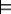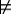## 14.2 Learning with Individuals and Relations

Learning in terms of individuals and relations allows for learning where the structure of relationships is important for prediction. The learning methods presented in Chapter 7 assumed that the feature values are meaningful; something useful could be predicted about a case by using the values of features. However, when the examples are about individuals and relations, the value of a property may be a meaningless name of an individual. It is only by considering the attributes of the individual that the name denotes, and its relationship to other individuals, that an agent is able produce meaningful predictions. The learned knowledge can also be applied to individuals that did not appear in the training set.

Because much of this work has been done in the context of logic programming, it is often called inductive logic programming.

Example 14.12: Suppose a trading agent the following data set, in terms of the individual-property-value triples, about which resorts a person likes:
 Individual Property Value joe likes resort_14 joe dislikes resort_35 ... ... ... resort_14 type resort resort_14 near beach_18 beach_18 type beach beach_18 covered_in ws ws type sand ws color white ... ... ...

The agent wants to learn what Joe likes. What is important is not the value of the likes property, which is just a meaningless name, but the properties of the individual denoted by the name. Feature-based representations cannot do anything with this data set beyond learning that Joe likes resort_14, but not resort_35.

The sort of theory the agent should learn is that Joe likes resorts near sandy beaches. This theory can be expressed as a logic program:

prop(joe,likes,R) ←
prop(R,type,resort) ∧
prop(R,near,B) ∧
prop(B,type,beach) ∧
prop(B,covered_in,S) ∧
prop(S,type,sand).

Logic programs provide the ability to be able to represent deterministic theories about individuals and relations. This rule can be applied to resorts and beaches that Joe has not visited.

The input to an inductive logic programming learner includes the following:

• A is a set of atoms whose definitions the agent is learning.
• E+ is the set of ground instances of elements of A, called the positive examples, that are observed to be true.
• E- is the set of ground instances of elements of A, called the negative examples, that are observed to be false.
• B, the background knowledge, is a set of clauses that define relations that can be used in the learned logic programs.
• H is a space of possible hypotheses. H is often represented implicitly as a set of operators that can generate the possible hypotheses. We assume that each hypothesis is a logic program.
Example 14.13: In Example 14.12, suppose the agent wants to learn what Joe likes. In this case, the inputs are
• A={prop(joe,likes,R)}
• E+={prop(joe,likes,resort_14),...}. For the example that follows, we assume there are many such items that Joe likes.
• E-={prop(joe,likes,resort_35),...}. Note that these are written in the positive form; they have been observed to be false.
• B={prop(resort_14,type,resort),prop(resort_14,near,beach_18),...}. This set contains all of the facts about the world that are not instances of A. The agent is not learning about these.
• H is a set of logic programs defining prop(joe,likes,R). The heads of the clauses unify with prop(joe,likes,R). H is too big to enumerate.

All of these, except for H, are given explicitly in the formulation of the problem.

The aim is to find a simplest hypothesis h∈H such that

B∧hE+ and
B∧hE-.

That is, the hypothesis implies the positive evidence and does not imply the negative evidence. It must be consistent with the negative evidence being false.

We, thus, want to find an element of the version space. This is similar to the definition of abduction, in which the knowledge base in abduction corresponds to the background knowledge. The hypothesis space of inductive logic programming is the set of logic programs. The second condition corresponds to consistency.

For the rest of this section, assume that there is a single target n-ary relation whose definition we want to learn, and for which we use the predicate symbol t. That is, A={t(X1,...,Xn)}. The hypothesis space consists of possible definitions for this relation using a logic program.

There are two main strategies used in inductive logic programming:

• The first strategy is to start with the simplest hypothesis and make it more complicated to fit the data. Because the logic program only states positive facts, the simplest hypothesis is the most general hypothesis, which is simply that t(X1,...,Xn) is always true. This hypothesis implies the positive examples, but it also implies the negative examples, if any exist. This strategy involves a general-to-specific search. It tries to find the simplest hypothesis that fits the data by searching the hypothesis space from the simplest hypothesis to more complex hypotheses, always implying the positive examples, until a hypothesis that does not imply the negative examples is found.
• The second strategy is to start with a hypothesis that fits the data and to make it simpler while still fitting the data. A hypothesis that fits the data is the set of positive examples. This strategy involves a specific-to-general search: start with the very specific hypothesis in which only the positive examples are true, and then generalize the clauses, avoiding the negative cases.

We will expand on the general-to-specific search for definite clauses. The initial hypothesis contains a single clause:

{t(X1,...,Xn) ←}.

A specialization operator takes a set G of clauses and returns a set S of clauses that specializes G. To specialize means that SG.

The following are three primitive specialization operators:

• Split a clause in G on condition c. Clause a←b in G is replaced by two clauses: a←b∧c and a←b∧¬c.
• Split a clause a←b in G on a variable X that appears in a or b. Clause a←b is replaced by the clauses
a ←b ∧X=t1.
...
a ←b ∧X=tk.

where the ti are terms.

• Remove a clause that is not necessary to prove the positive examples.

The last operation changes the predictions of the clause set. Those cases no longer implied by the clauses are false. These primitive specialization operators are used together to form the operators of H. The operators of H are combinations of primitive specialization operations designed so that progress can be evaluated using a greedy look-ahead. That is, the operators are defined so that one step is adequate to evaluate progress.

The first two primitive specialization operations should be carried out judiciously to ensure that the simplest hypothesis is found. An agent should carry out the splitting operations only if they make progress. Splitting is only useful when combined with clause removal. For example, adding an atom to the body of a clause is equivalent to splitting on the atom and then removing the clause containing the negation of the atom. A higher-level specialization operator may be to split on the atom prop(X,type,T) for some variable X that appears in the clause, split on the values of T, and remove the resulting clauses that are not required to imply the positive examples. This operator makes progress on determining which types are useful.

1: Procedure TDInductiveLogicProgram(t,B,E+,E-,R)
2:           Inputs
3:                     t: an atom whose definition is to be learned
4:                     B: background knowledge is a logic program
5:                     E+: positive examples
6:                     E-: negative examples
7:                     R: set of specialization operators
8:           Output
9:                     logic program that classifies E+ positively and E- negatively or if no program can be found
10:           Local
11:                     H is a set of clauses
12:           H←{t(X1,...,Xn)←}
13:           while (there is e∈E- such that B∪He)
14:                     if (there is r∈R such that B∪r(H)E+) then
15:                               Select r∈R such that B∪r(H)E+
16:                               H ←r(H)
17:                     else
18:                               return
19:
20:
21:           return H
Figure 14.1: Top-down induction of a logic program

Figure 14.1 shows a local search algorithm for the top-down induction of a logic program. It maintains a single hypothesis that it iteratively improves until finding a hypothesis that fits the data or until it fails to find such a hypothesis.

At each time step it chooses an operator to apply with the constraint that every hypothesis entails the positive examples. This algorithm glosses over two important details:

• which operators to consider and
• which operator to select.

The operators should be at a level so that they can be evaluated according to which one is making progress toward a good hypothesis. In a manner similar to decision-tree learning, the algorithm can perform a myopically optimal choice. That is, it chooses the operator that makes the most progress in minimizing the error. The error of a hypothesis can be the number of negative examples that are implied by the hypothesis.

Example 14.14: Consider Example 14.12, in which the agent must learn about Joe's likes and dislikes. The first hypothesis is that Joe likes everything:
{prop(joe,likes,R)←},

which is inconsistent with the negative evidence.

It can split on properties that contain the individuals that Joe likes. The only way for the specialization to make progress - to prove fewer negative examples while implying all positive examples - is to contain the variable R in the body. Thus, it must consider triples in which R is the subject or the object.

• It could consider splitting on the property type, splitting on the value of type, and keeping only those that are required to prove the positive examples. This results in the following clause (assuming the positive examples only include resorts):
{prop(joe,likes,R)←prop(R,type,resort)}.

There can be other clauses if the positive examples include things other than resorts that Joe likes. If the negative examples include non-resorts, this split would be useful in reducing the error.

• It could consider splitting on other properties that can be used in proofs of the positive examples, such as near, resulting in
{prop(joe,likes,R)←prop(R,near,B)}

if all of the positive examples are near something. If some of the negative examples are not near anything, this specialization could be useful in reducing the error.

It could then choose whichever of these splits makes the most progress. In the next step it could add the other one, other properties of R, or properties of B.